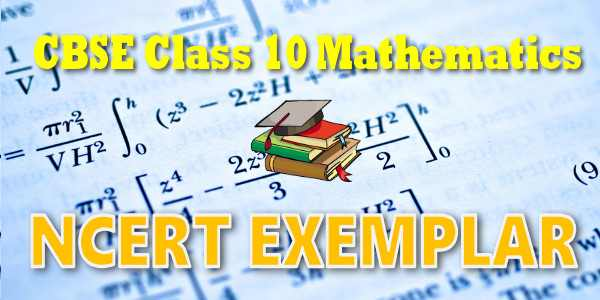No products in the cart.

# NCERT Exemplar Solutions for Mathematics Triangles

## NCERT Exemplar Solutions for Mathematics Triangles### Student Subscription

##### Unlock the exclusive content designed for the toppers

We will update content for this category shortly. Please visit this category after few days or subscribe to our newsletter by email for latest updates

### NCERT Exemplar Problem book and Solutions for Class 10th Mathematics

NCERT exemplar problem book and Solutions Class 10 Maths PDF (Download) Free from myCBSEguide app and myCBSEguide website. Ncert solution class 10 Maths includes text book solutions. NCERT Solutions for CBSE Class 10 Maths have total 15 chapters. Class 10 Maths ncert exemplar problem and Solutions in pdf for free Download are given in this website. Ncert Maths class 10 exemplar solutions PDF and Maths ncert class 10 PDF exemplar problems and solutions with latest modifications and as per the latest CBSE syllabus are only available in myCBSEguide.

## CHAPTER SIX TRIANGLES

• 6.1 Similar figures
• 6.2 Similarity of triangles: (Prove) If a line is drawn parallel to one side of a triangle to intersect the other two sides in distinct points, the other two sides are divided in the same ratio
• 6.3 (Motivate) If a line divides two sides of a triangle in the same ratio, the line is parallel to the third side.”
• 6.4 Basic Proportionality Theorem
• 6.5 Criteria for similarity of triangles
• 6.6 Area of similar triangles
• 6.7 Pythagoras theorem
• 6.8 Converse of Pythagoras theorem

## NCERT Exemplar Problems & Solutions## myCBSEguide

Trusted by 1 Crore+ Students## Test Generator

Create papers online. It's FREE.## CUET Mock Tests

75,000+ questions to practice only on myCBSEguide app